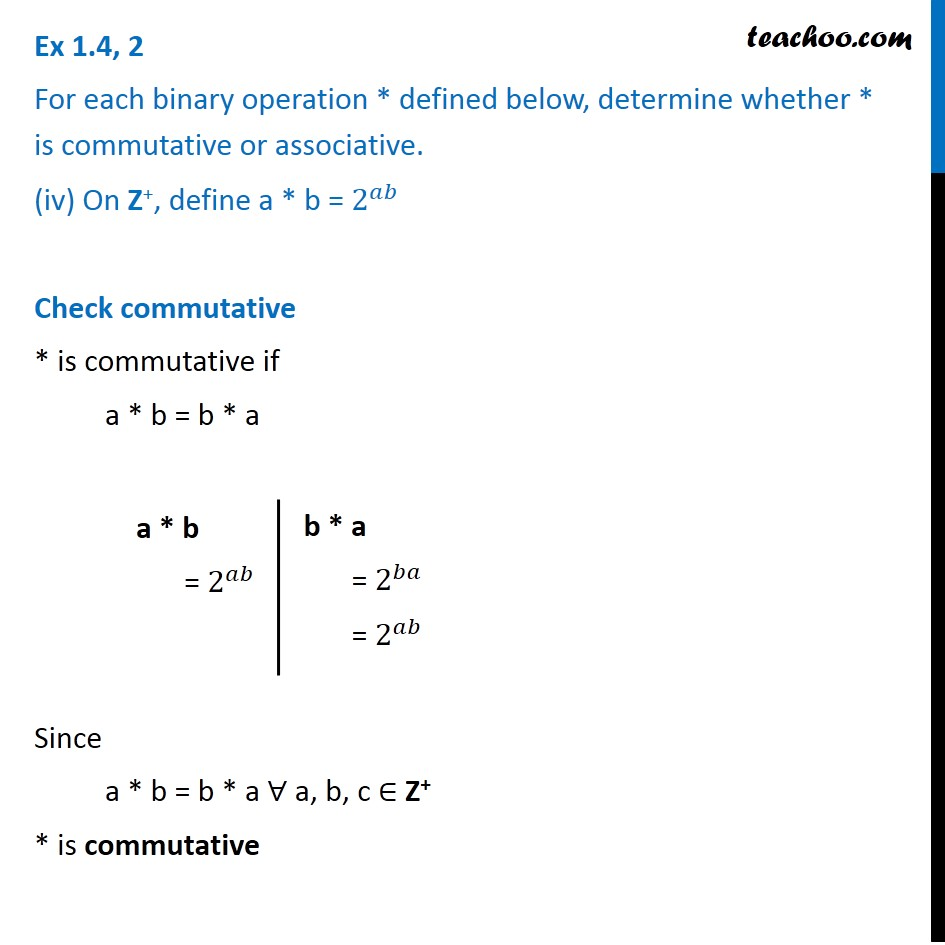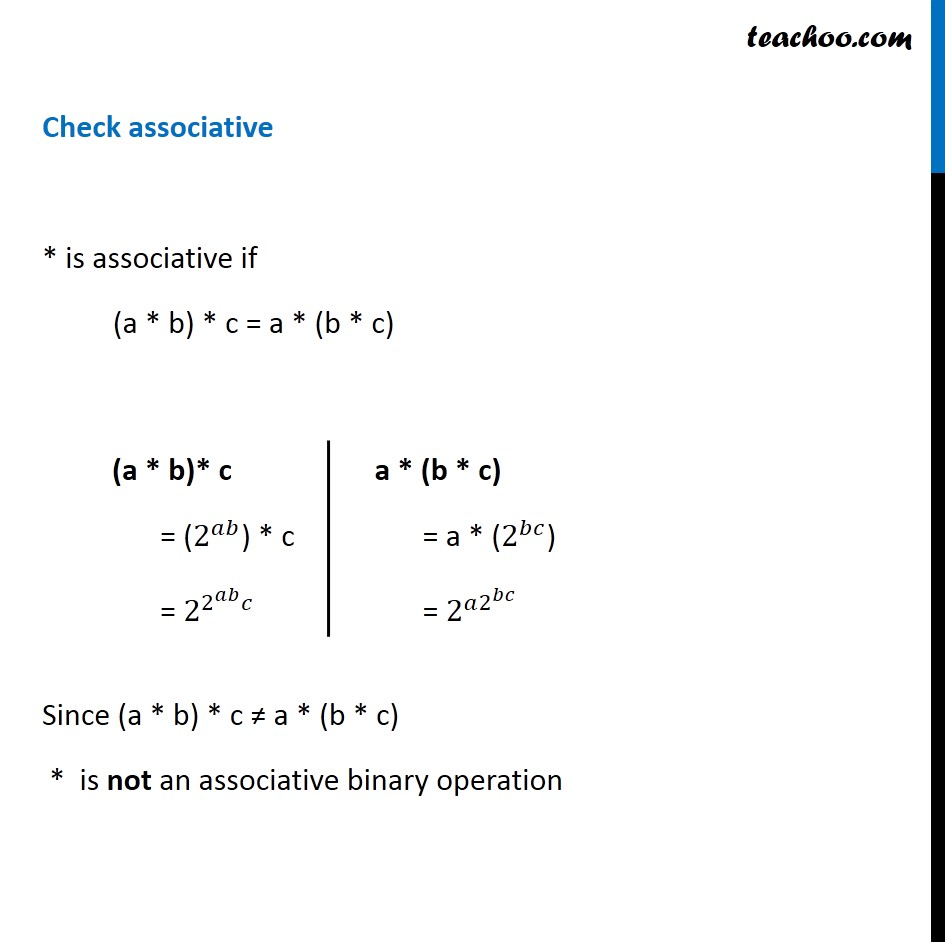Binary Operations

Chapter 1 Class 12 Relation and Functions
Serial order wiseLearn in your speed, with individual attention - Teachoo Maths 1-on-1 Class

### Transcript

Ex 1.4, 2 For each binary operation * defined below, determine whether * is commutative or associative. (iv) On Z+, define a * b = 2^𝑎𝑏 Check commutative * is commutative if a * b = b * a Since a * b = b * a ∀ a, b, c ∈ Z+ * is commutative a * b = 2^𝑎𝑏 b * a = 2^𝑏𝑎 = 2^𝑎𝑏 Check associative * is associative if (a * b) * c = a * (b * c) Since (a * b) * c ≠ a * (b * c) * is not an associative binary operation (a * b)* c = (2^𝑎𝑏) * c = 2^(2^𝑎𝑏 𝑐) a * (b * c) = a * (2^𝑏𝑐) = 2^(𝑎2^𝑏𝑐 )